# ISEE Upper Level Quantitative : Rhombuses

## Example Questions

### Example Question #1 : Rhombuses

In Rhombus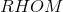,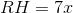and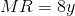. Which is the greater quantity?

(A)(B)(a) and (b) are equal

(b) is the greater quantity

It cannot be determined which of (a) and (b) is greater

(a) is the greater quantity

(a) is the greater quantity

Explanation:

The four sides of a rhombus, by defintion, have equal length, so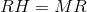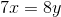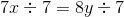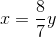Sinceandare positive,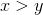.

### Example Question #61 : Quadrilaterals

A rhombus has diagonals of length two and one-half feet and six feet. Which is the greater quantity?

(A) The perimeter of the rhombus

(B) Four yards

(B) is greater

It is impossible to determine which is greater from the information given

(A) is greater

(A) and (B) are equal

(A) is greater

Explanation:

It will be easier to look at these measurements as inches for the time being: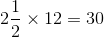and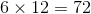, so these are the lengths of the diagonals in inches.

The diagonals of a rhombus are each other's perpendicular bisector, so, as can be seen in the diagram below, one side of a rhombus and one half of each diagonal form a right triangle. If we letbe the length of one side of the rhombus, then this is the hypotenuse of that right triangle; its legs are one-half the lengths of the diagonals, or 15 and 36 inches.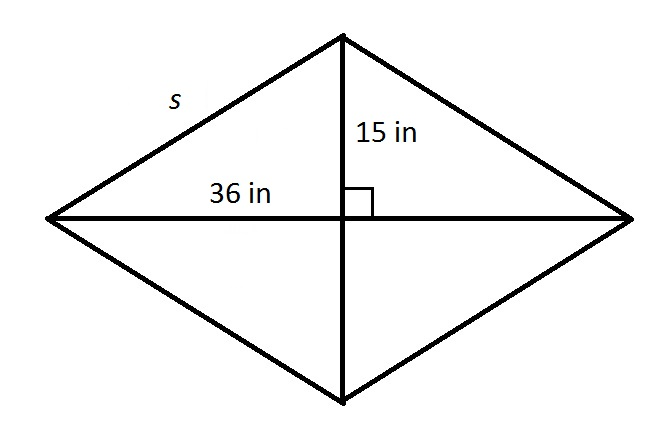By the Pythagorean Theorem,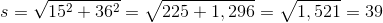Each side of the rhombus measures 39 inches, and its perimeter is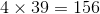inches.

Four yards is equal to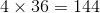inches, so (A) is greater.

### Example Question #62 : Quadrilaterals

A rhombus has sidelength ten inches; one of its diagonals is one foot long. Which is the greater quantity?

(a) The length of the other diagonal

(B) One and one-half feet

It is impossible to determine which is greater from the information given

(A) is greater

(B) is greater

(A) and (B) are equal

(B) is greater

Explanation:

The diagonals of a rhombus are each other's perpendicular bisector, so, as can be seen in the diagram below, one side of a rhombus and one half of each diagonal form a right triangle. If the other diagonal has length, then the right triangle has hypotenuse 10 inches and legs one-half of one foot and- that is, six inches and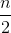.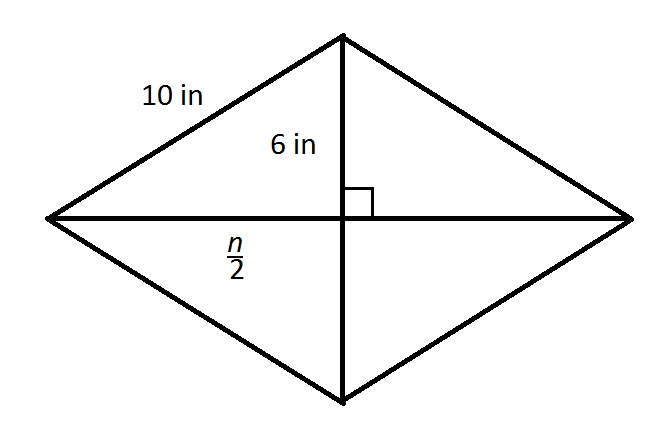This triangle fits the well-known Pythagorean triple of 6-8-10, so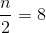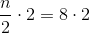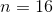The other diagonal measures 16 inches. One and one-half feet is equal to 18 inches, making (B) greater.

### Example Question #4 : Rhombuses

Rhombushas two diagonals that intersect at point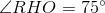. Which is the greater quantity?

(a)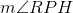(b)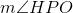(b) is greater

(a) and (b) are equal

It is impossible to tell from the information given

(a) is greater

The diagonals of a rhombus always intersect at right angles, so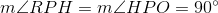. The measures of the interior angles of the rhombus are irrelevant.